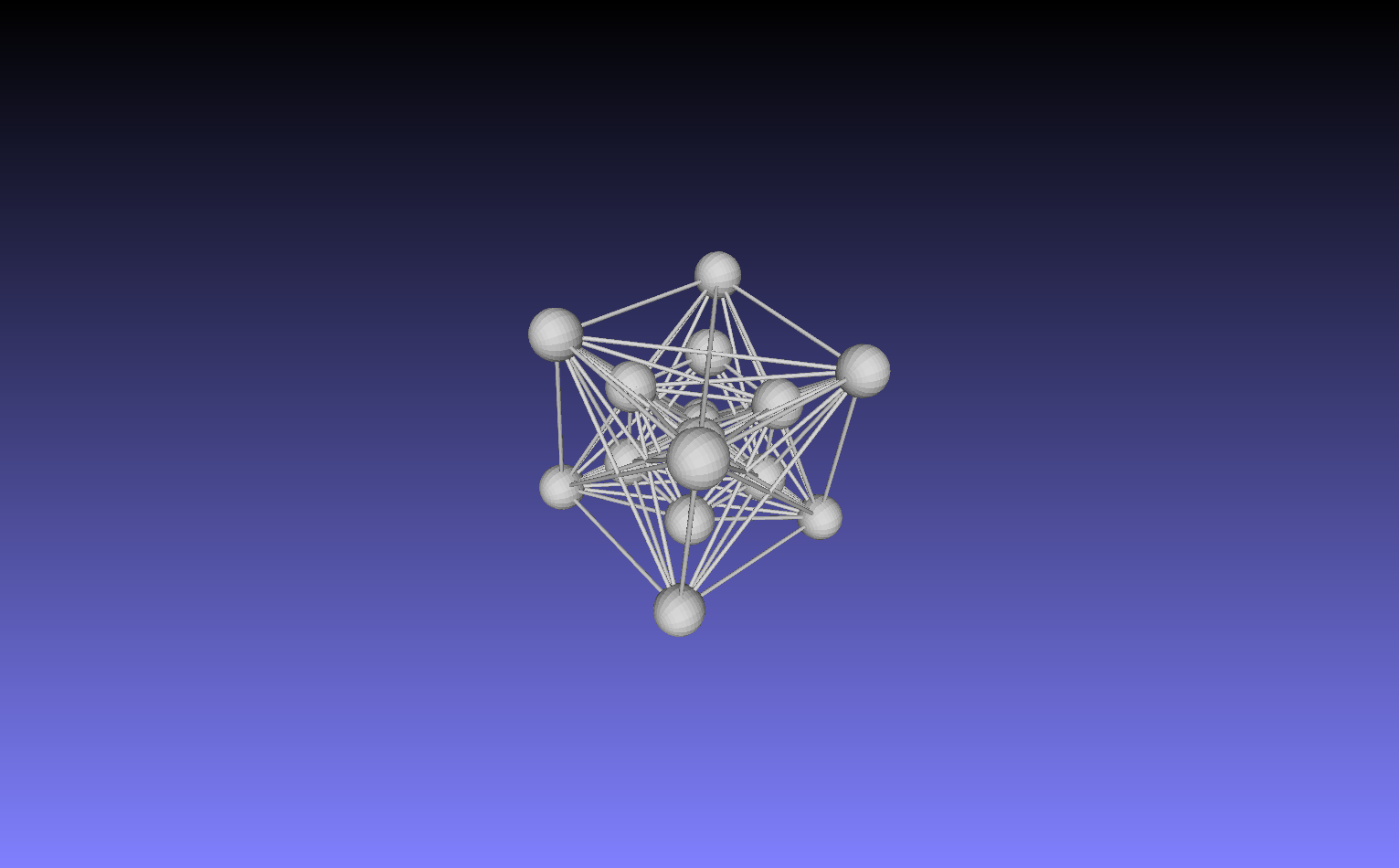# Latest Paper – Unimodular rotation of E8 to H4 600-cells

## Please see my latest paper that describes some advances in understanding the E8 to H4 rotation matrix

https://theoryofeverything.org/TOE/JGM/Unimodular-Rotation-of-E8-to-H4.pdf

Abstract: We introduce a unimodular Determinant=1 8×8 rotation matrix to produce four 4 dimensional copies of H4 600-cells from the 240 vertices of the Split Real Even E8 Lie group. Unimodularity in the rotation matrix provides for the preservation of the 8 dimensional volume after rotation, which is useful in the application of the matrix in various fields, from theoretical particle physics to 3D visualization algorithm optimization.

# Stereoscopic 3D Interactive Solar system simulation (shoemaker-Levy-9)

## It requires local installation of the free Mathematica CDF plugin.

This now includes the 1998 OR2 “Planet Killer” that will pass very near Earth in April 2020 (when selecting the Asteroid vs. Comet).

Now iwht improved UI, better planet scaling, and Anaglyph viz.

# Mathematica Analysis of Cohl Furey’s octonion and Clifford group theoretic ℂ⊗O assignments to standard model particles

I’ve read some recent papers by Cohl Furey and was intrigued by the potential relationship between the octonion and Clifford group theoretic assignments to standard model particles. Since I have developed an extensive Mathematica notebook to perform symbolic analysis using these structures (derived primarily for E8 Lie group work), I decided to follow her suggestion… “The reader is encouraged to check that C⊗O forms the 64-complex-dimensional Clifford algebra Cl(6), generated by the set {i e1,…i e6} acting on f”.

So to that end, I created the this .pdf with some preliminary results of that analysis. I found a few minor issues, but so far the model seems to symbolically compute consistently. Very cool!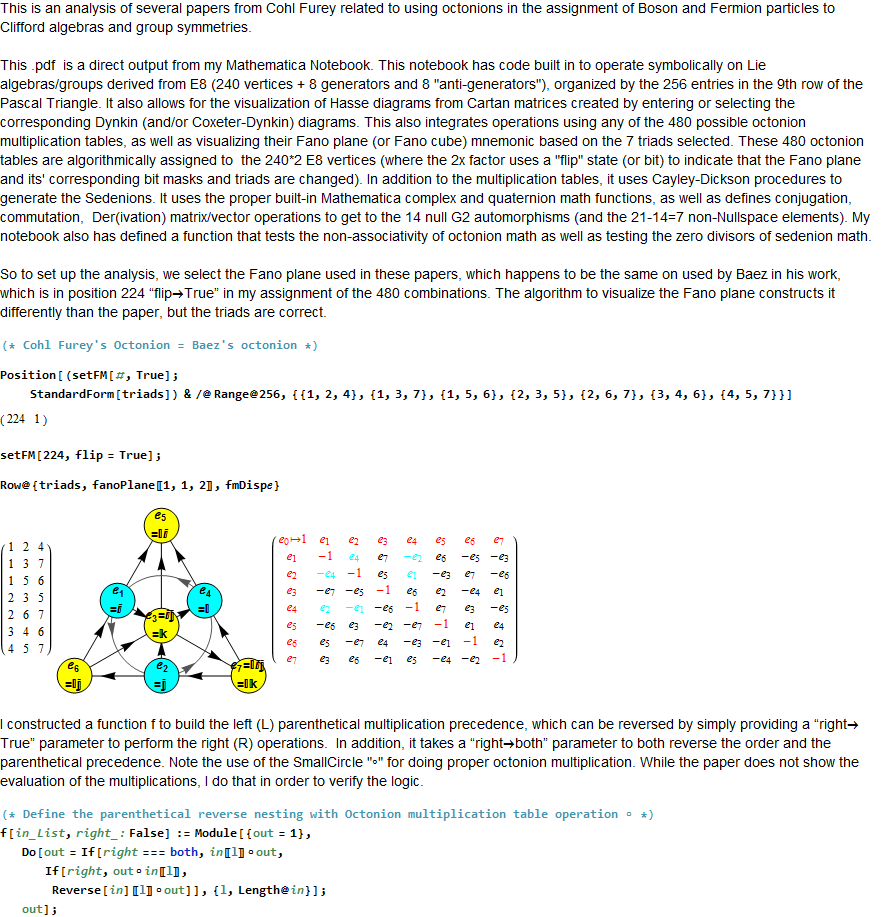# Latest paper: Even FibBinary Numbers and the Golden Ratio

Please see my latest paper here.

It adds information related to my previous blog post here.

Abstract: Previously, a determination of the relationship between the Natural numbers (N) and the n’th odd fibbinary number has been made using a relationship with the Golden ratio phi=(Sqrt+1}/2 and tau=1/phi. Specifically, if the n’th odd fibbinary equates to the j’th N, then j=Floor[n phi^2 – 1]. This note documents the completion of the relationship for the even fibbinary numbers, such that if the n’th even fibbinary equates to the j’th N, then j=Floor[n phi]-1, starting at j=0 for n=1. Alternatively, starting at j=2 for n=1, then j=Floor[n phi + tau].

Title:Even FibBinary Numbers and the Golden Ratio
Authors: J Gregory Moxness
Comments: Dec 31 2018, 5 pages, 4 Figures.
Subjects: Combinatorics

Below is a listing of the N integers represented by their 38 odd FibBinaries, along with the binary and Gray code Compositions.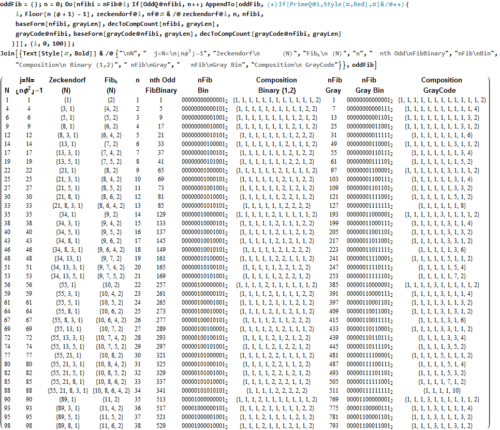Below is a listing of the first 63 N integers represented by their even FibBinaries, along with the binary and Gray code Compositions.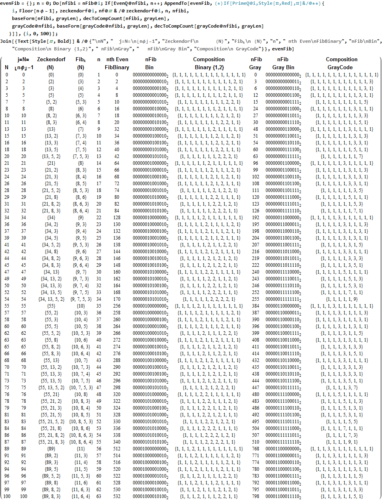Code output examples:Mathematica Code Snippets: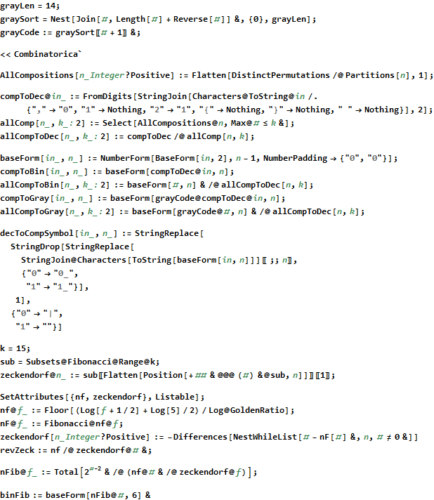# Integer Compositions, Gray Code , and the Fibonacci Sequence

I was prompted by Chris Birke to take a look at this very interesting paper by Linus Lindroos. Chris is working with my E8 to H4 Folding Matrix for optimizing computation using network theory and hyper-dimensional geometric structures.

I was able to validate the paper with some quick Mathematica code.

Below is the code that sets up the generation of the tables, including various binary, numeric, and symbolic (graph) Compositions.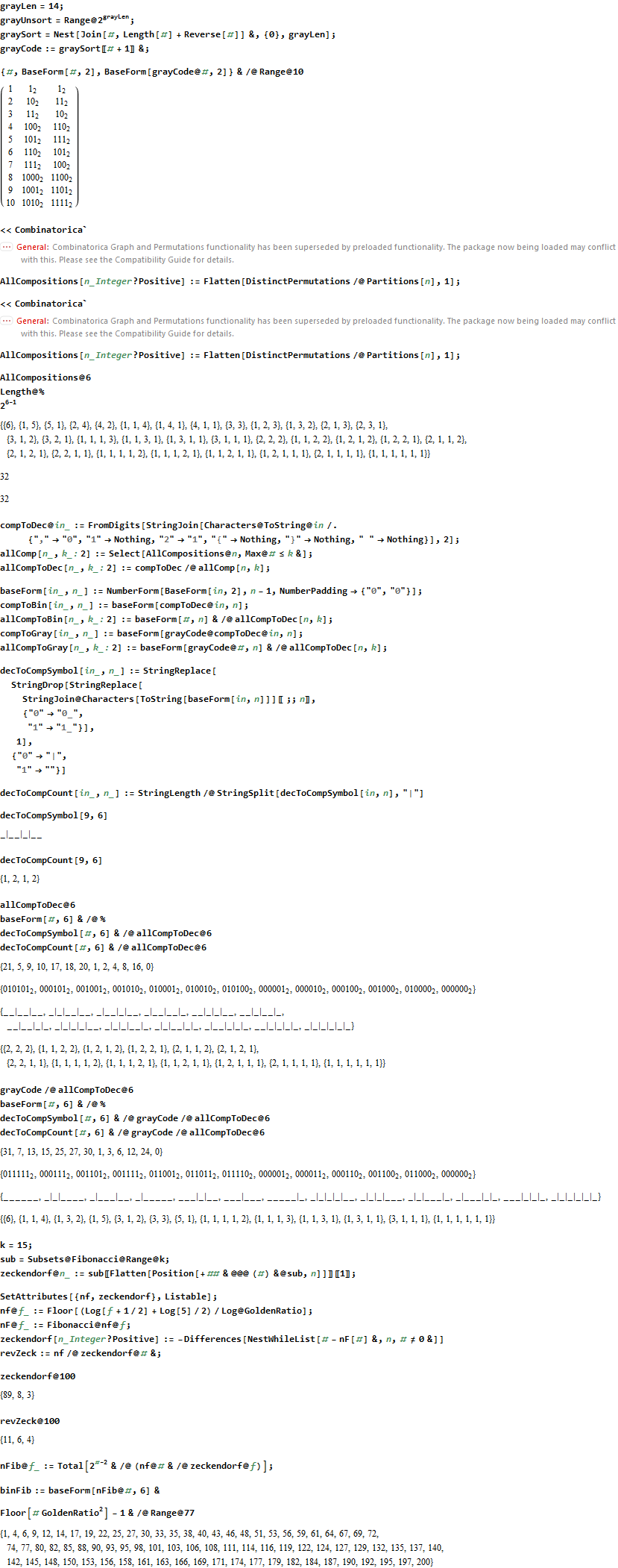Notice the last statement (above) lists the odd FibBinaries (up to N=200) as related to the Floor of the n*Phi^2-1, with n=1 to 77.

Below is a listing of the first 100 N integers represented by their odd and even FibBinaries, along with the binary and Gray code Compositions.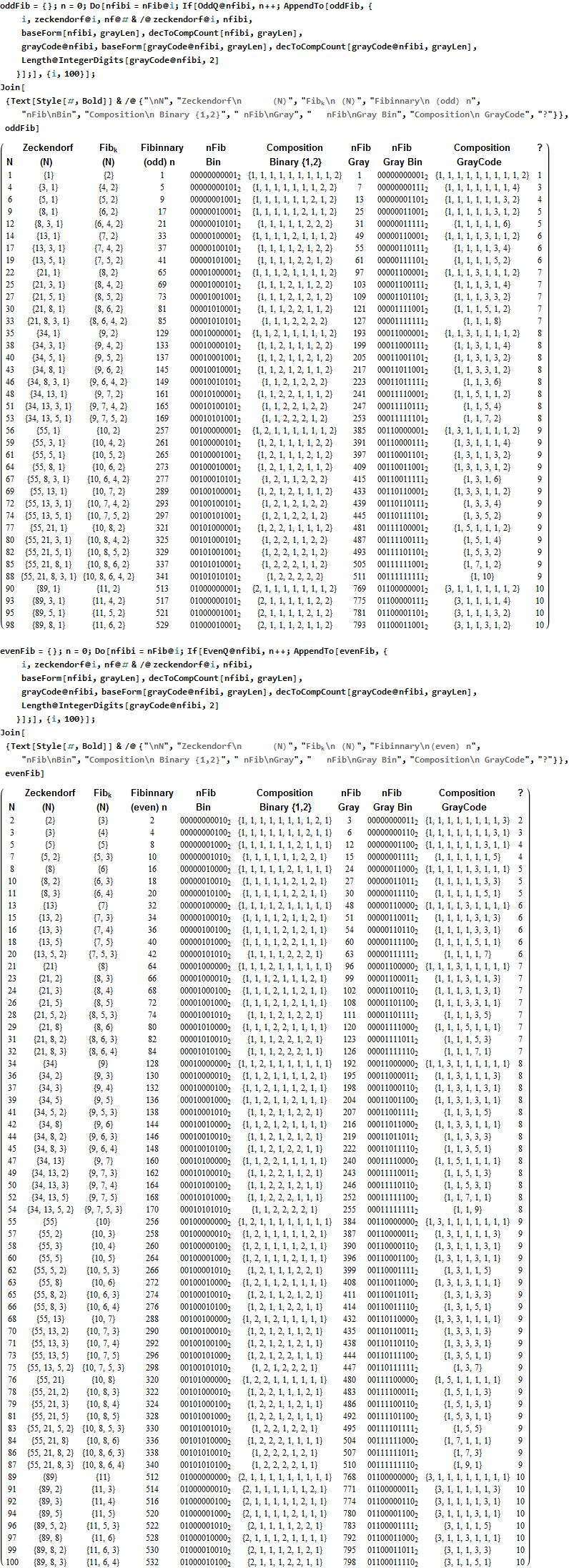# Working with E8+++

I wanted to confirm some work being done with E11 (or more specifically the Extended E8+++), so I used my “VisibLie” notebook (which includes the “SuperLie” package for analyzing Lie Algebras) to get the following information:

I first created the Dynkin diagram which produces the Cartan Matrix: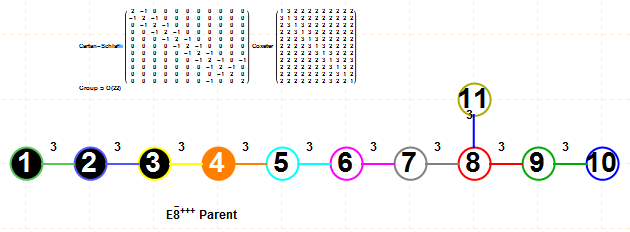This allows the evaluation of the EigenSystem of the Cartan matrix as follows: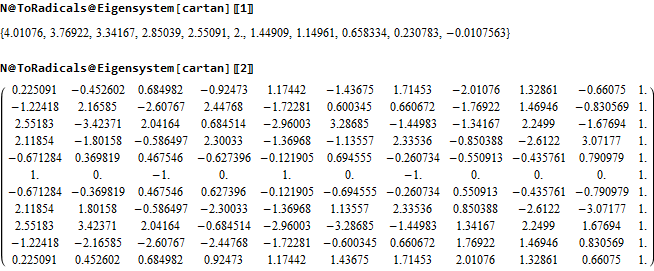As well as using the SuperLie package to evaluate the Positive Roots, Weights and Heights of the E8+++ (The first 80 are shown. For the full list in .pdf click here or click on the image below):The 4870 positive roots up to height 47 generate a Hasse diagram as follows (with 3 sections zoomed in):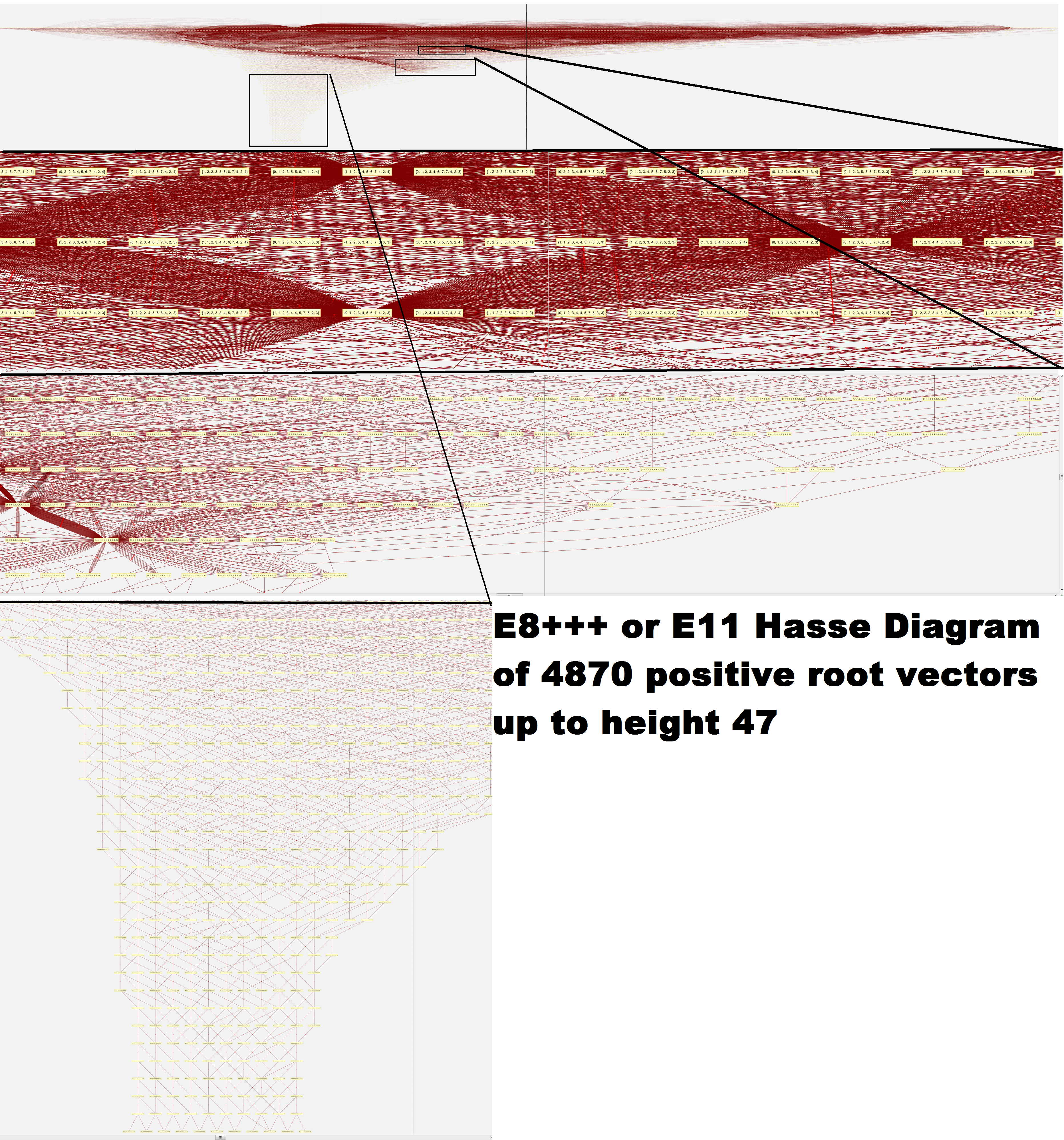I am working on replication of the Petrie projection by A.G. Lisi based on his prescription using his basis vectors for 2D projection and a Simple Roots Matrix: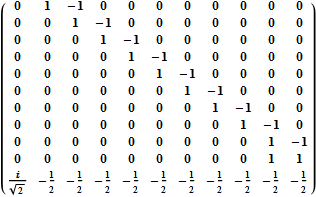Adjusting this E11 Simple Roots Matrix (srmE11) to match the Dynkin diagram above and then verifying it creates the same Cartan matrix, we have: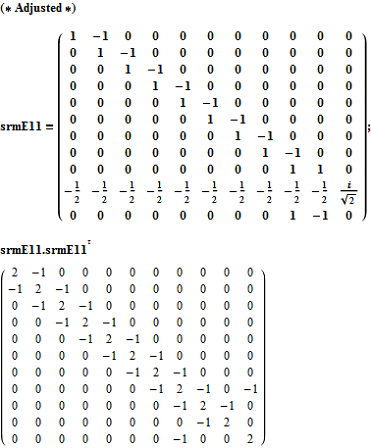To get the 9740 E8+++ vertices, we simply use the dot product of the transpose of srmE11 against the positive and negative root vectors (first 50 shown):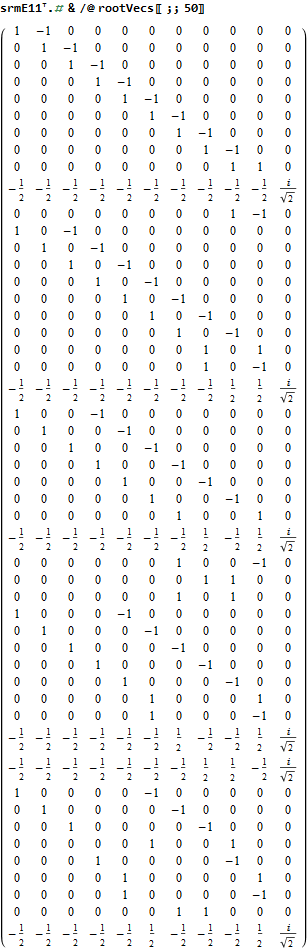A first cut at plotting these involves projecting them to a 2D using the dot product of the 2 basis vectors provided, which gives the following ListPlot: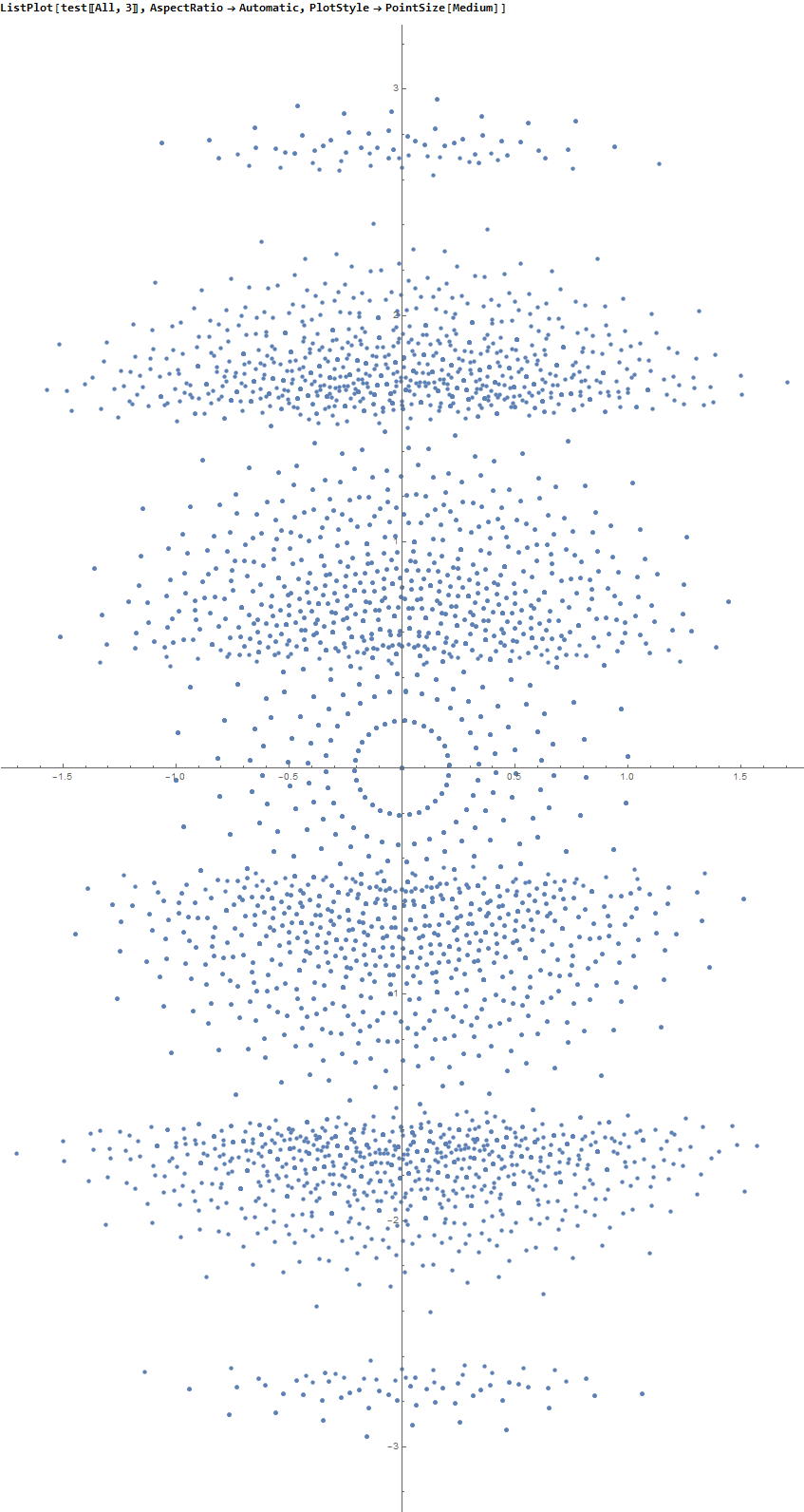The limited results from SuperLie are consistent with the image given by Lisi on the Bee’s BackReaction blog, after eliminating the vertical “levels” in my raw plot.

Email me if you want more detail, see errors, or would like to help.

Greg
JGMoxness@theoryofeverything.org

# Mapping the fourfold H4 600-cells emerging from E8: A mathematical and visual study

My latest paper is now available here or on Vixra.

{abstract}
It is widely known that the E8 polytope can be folded into two Golden Ratio (Φ) scaled copies of the 4 dimensional (4D) 120 vertex 720 edge H4 600-cell. While folding an 8D object into a 4D one is done by applying the dot product of each vertex to a 4×8 folding matrix, we use an 8×8 rotation matrix to produce four 4D copies of H4 600-cells, with the original two left side scaled 4D copies related to the two right side 4D copies in a very specific way. This paper will describe and visualize in detail the specific symmetry relationships which emerge from that rotation of E8 and the emergent fourfold copies of H4. It will also introduce a projection basis using the Icosahedron found within the 8×8 rotation matrix. It will complete the detail for constructing E8 from the 3D Platonic solids, Icosians, and the 4D H4 600-cell. Eight pairs of Φ scaled concentric Platonic solids are identified directly using the sorted and grouped 3D projected vertex norms present within E8. Finally, we will show the relationship of the Beordijk-Coxeter Tetrahelix emerging from the Petrie projection’s concentric rings of 30 vertices of the H4 600-cells.

This paper builds off of some recent work here, and here.

Below are a few figures from that paper.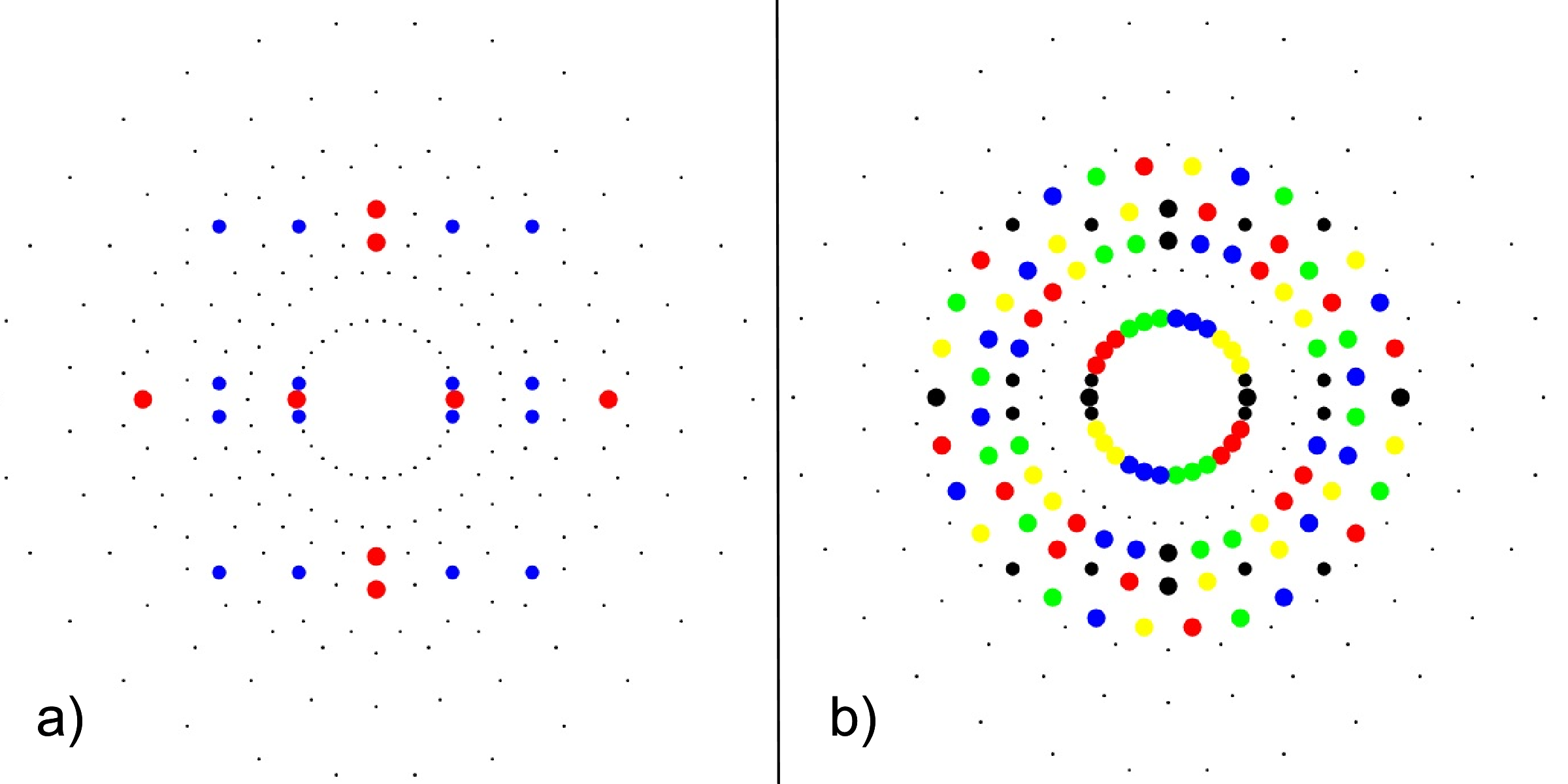FIG. 3: a) 24-cell highlighting the 16-cell (red on-axis vertices) and 8-cell (blue off-axis vertices), b) Snub 24-cell highlighting four pi/5 rotations of the 24-cell (black) in red, green, blue, yellow.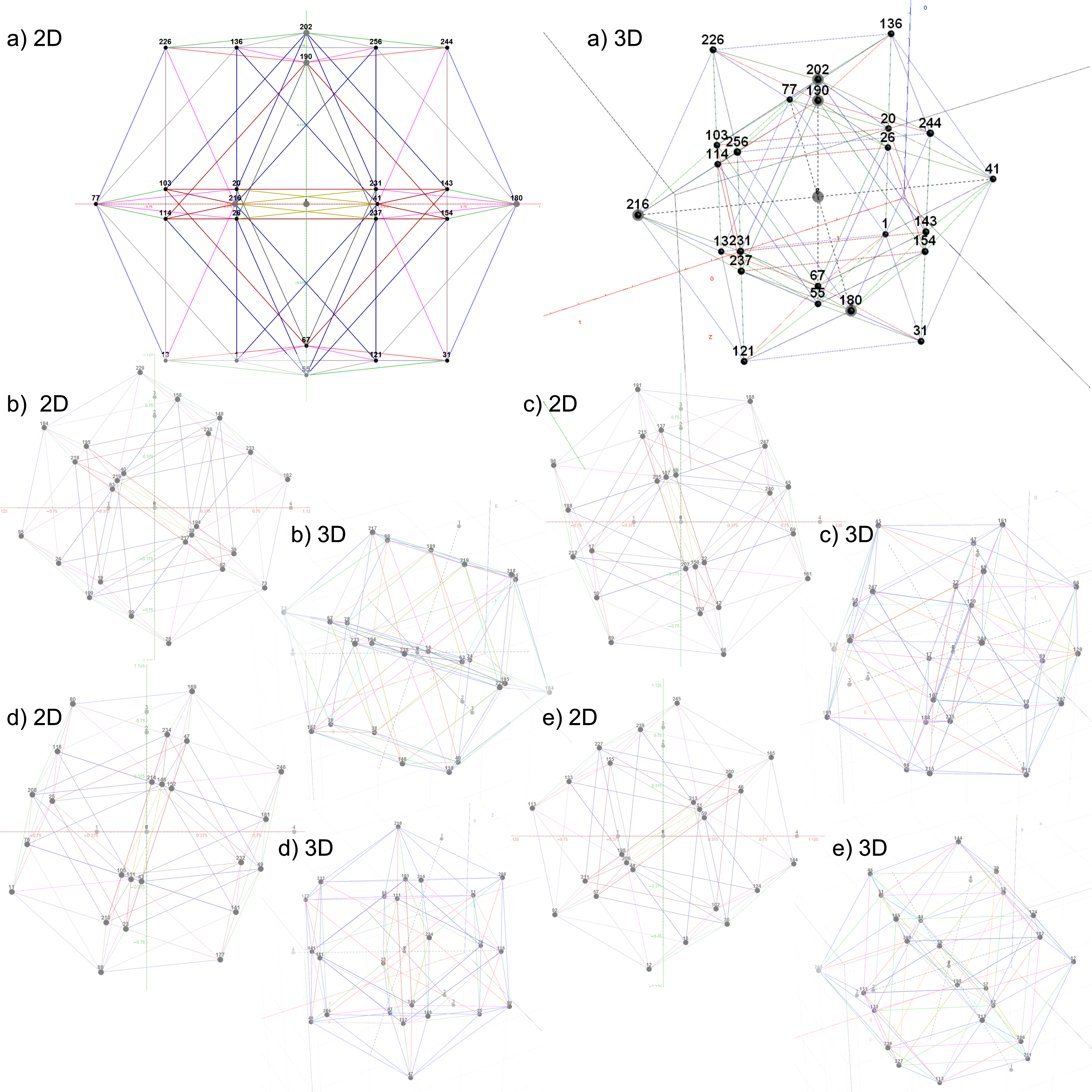FIG. 4: The 5 24-cell decomposition of one 600-cell, each with 2D and 3D vertex numbered projections including edges; a)
24-cell, b-e) Snub 24-cells from four pi/5 rotations of the 24-cell in a)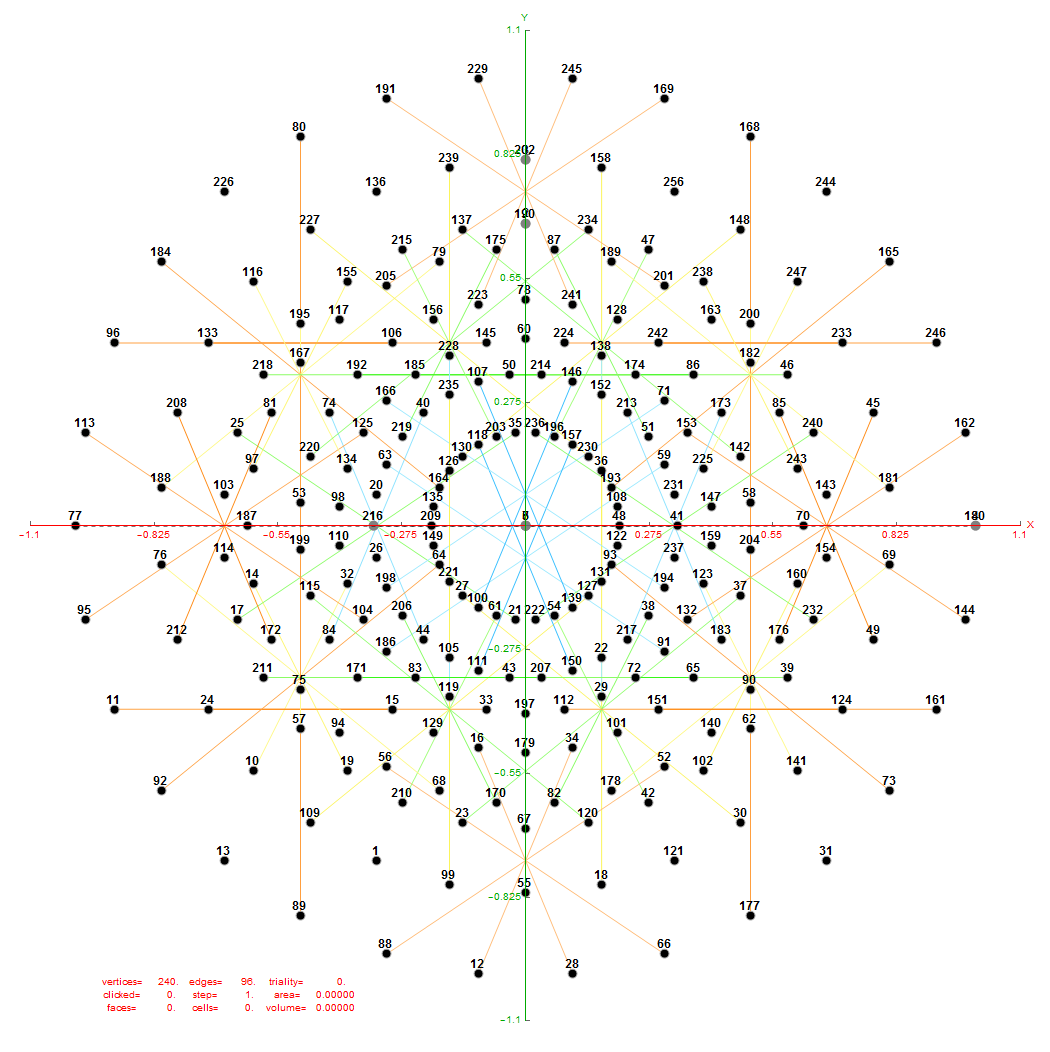FIG. 6: Vertex numbered Petrie projection of the rotated E8, showing the 96 edges of 8D norm l = 2Φ which links the Snub 24-cell H4 and H4Φ (L<->R) vertices (Note: the 8 excluded positive E8 8-Orthoplex (or equivalently, the H4 and H4Φ 4-Orthoplex) “generator vertices” are shown as larger gray labeled axis dots which overlap their darker black E8 vertices)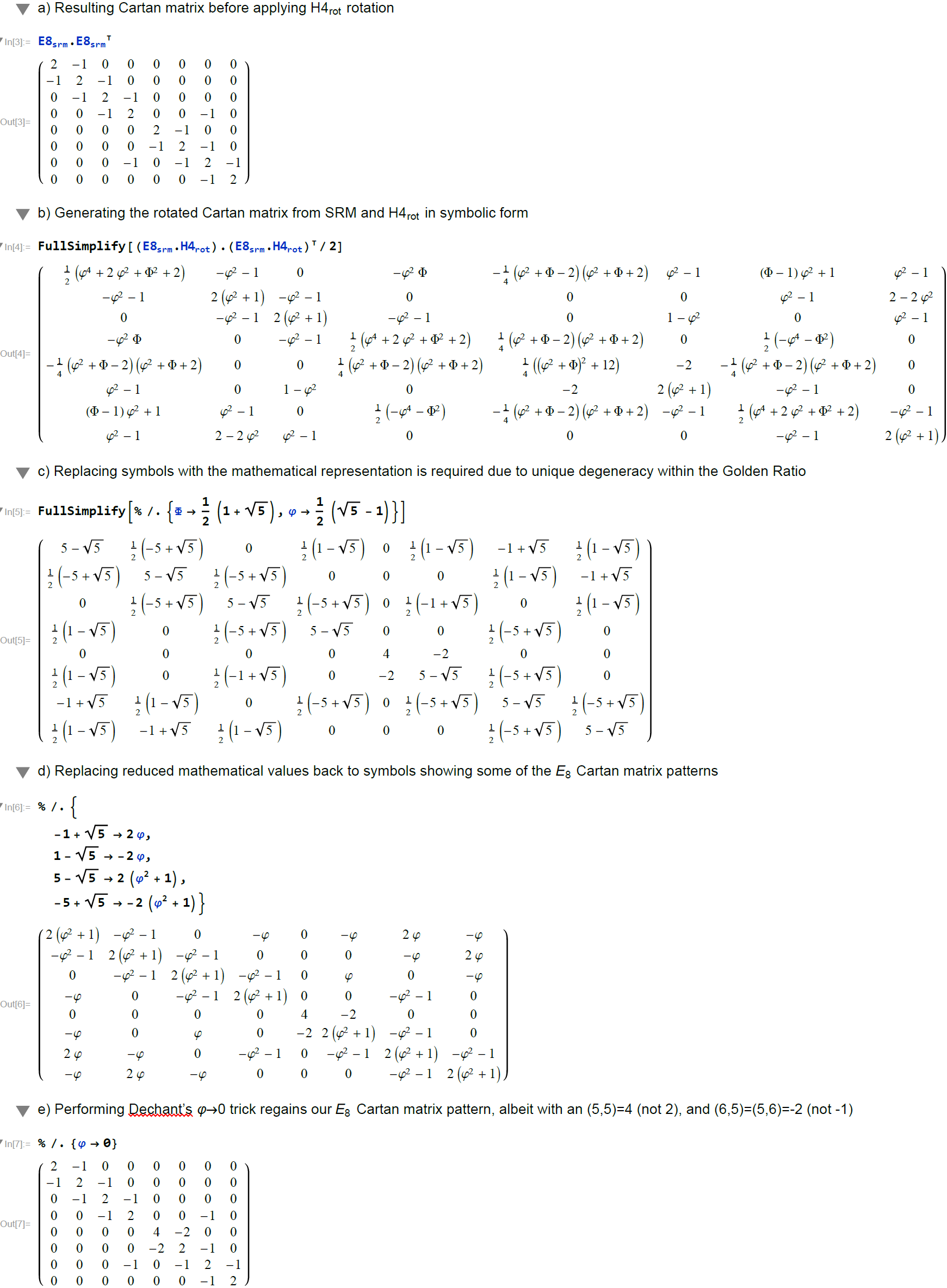FIG. 7: Symbolic analysis using MathematicaTM comparing the Cartan matrix before and after rotating the simple roots matrix (E8srm) used to create it (Note: the resulting Cartan matrix is not precisely that of E8, even after applying the Dechant’s Φ=0 trick)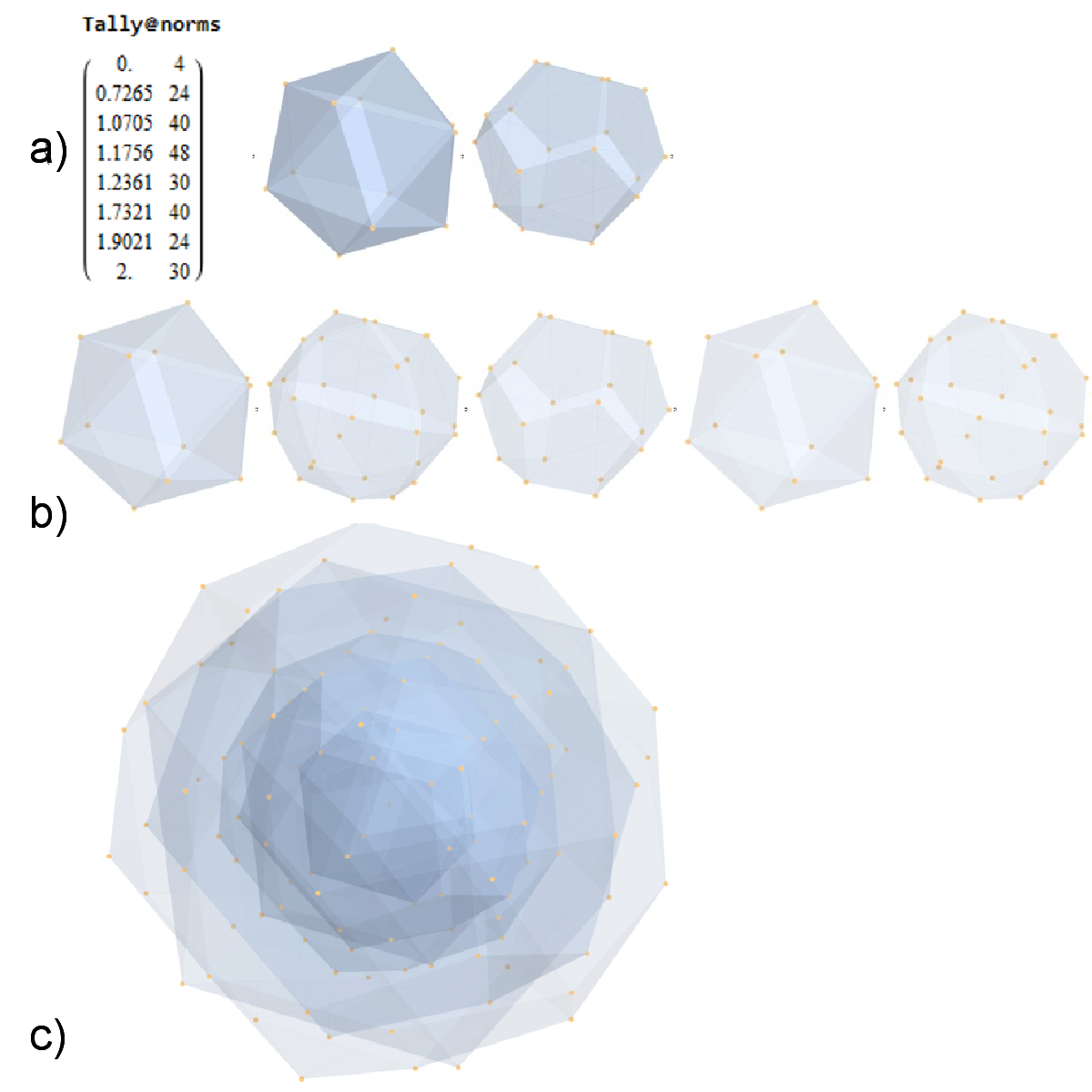FIG. 8: a) Sorted list of the vertex norms with their grouped vertex counts. b) 3D surface models for each of the 7 hulls of vertices c) Combined 3D surface model with increasing transparency for each successive hull.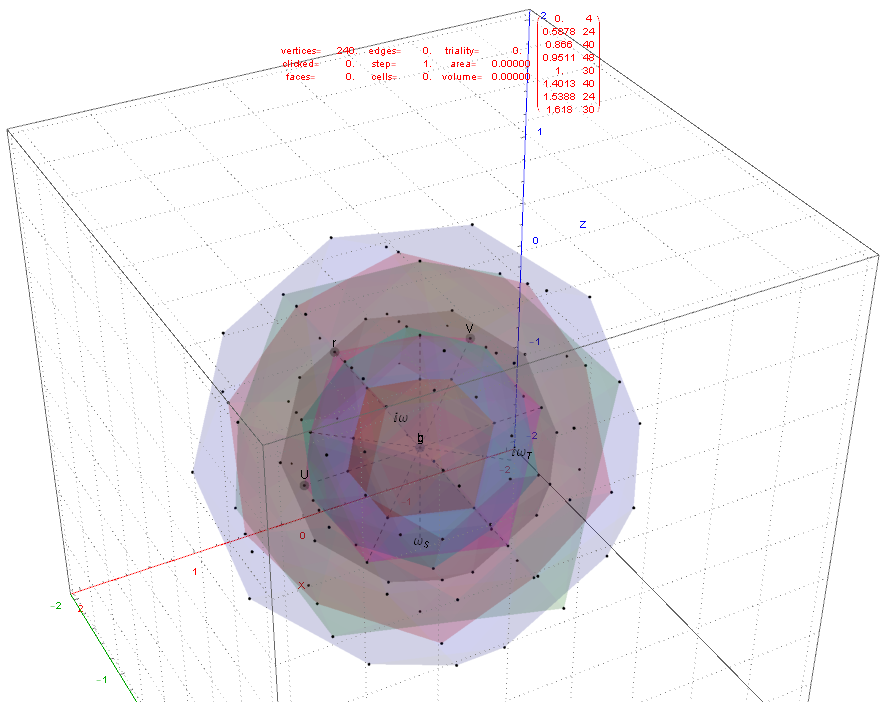FIG. 11: 3D projection using an orthonormal basis on H4 and H4Φ vertices (i.e. the rotated E8)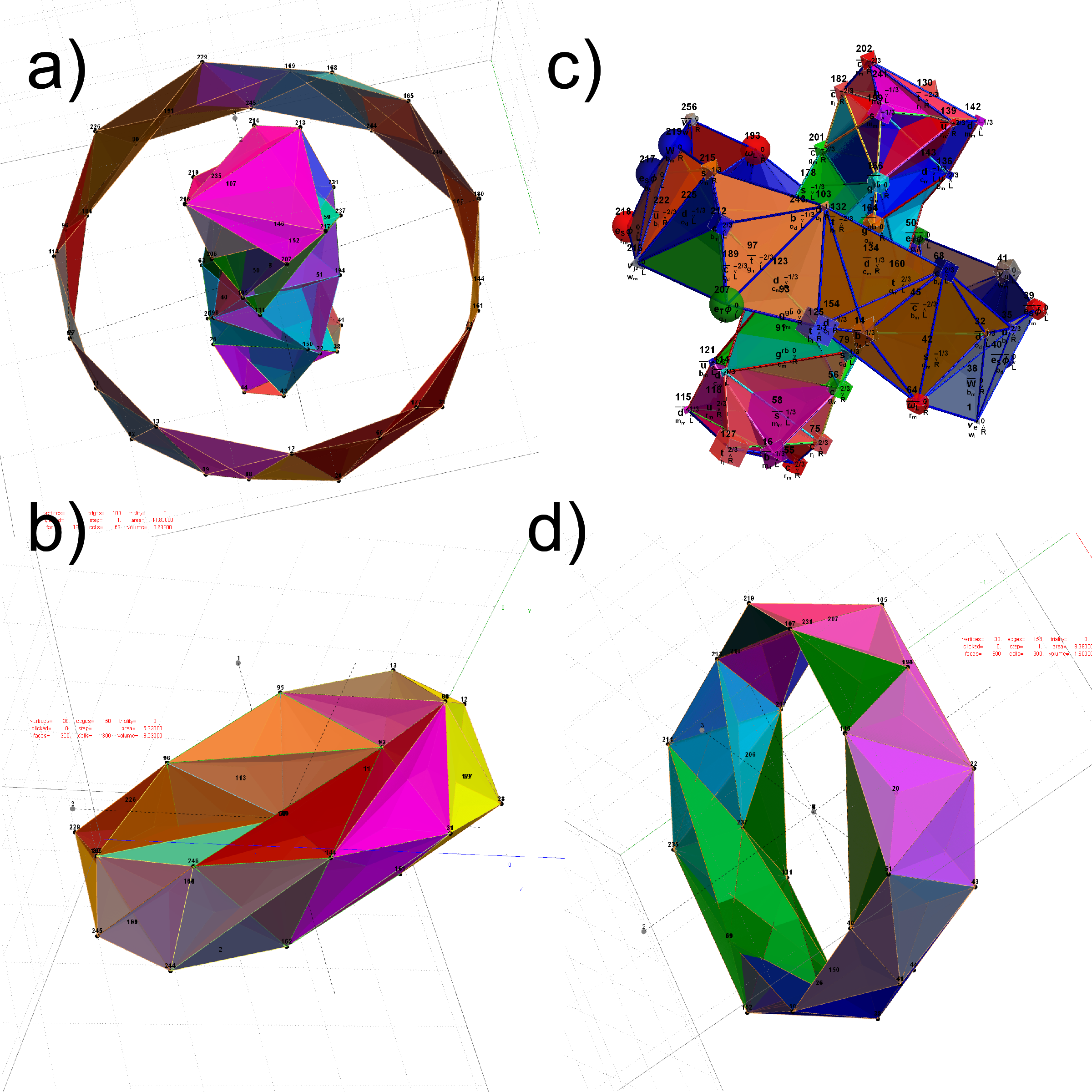FIG. 12: The Beordijk-Coxeter Tetrahelix emerging from the 8 concentric rings of 30 E8 and H4+H4Φ Petrie projection vertices; a) H4 inner and outer ring in 3D, b) with vertex size, shape, color assigned from physics model, c-d) inner and outer ring in orthonormal projection of H4Φ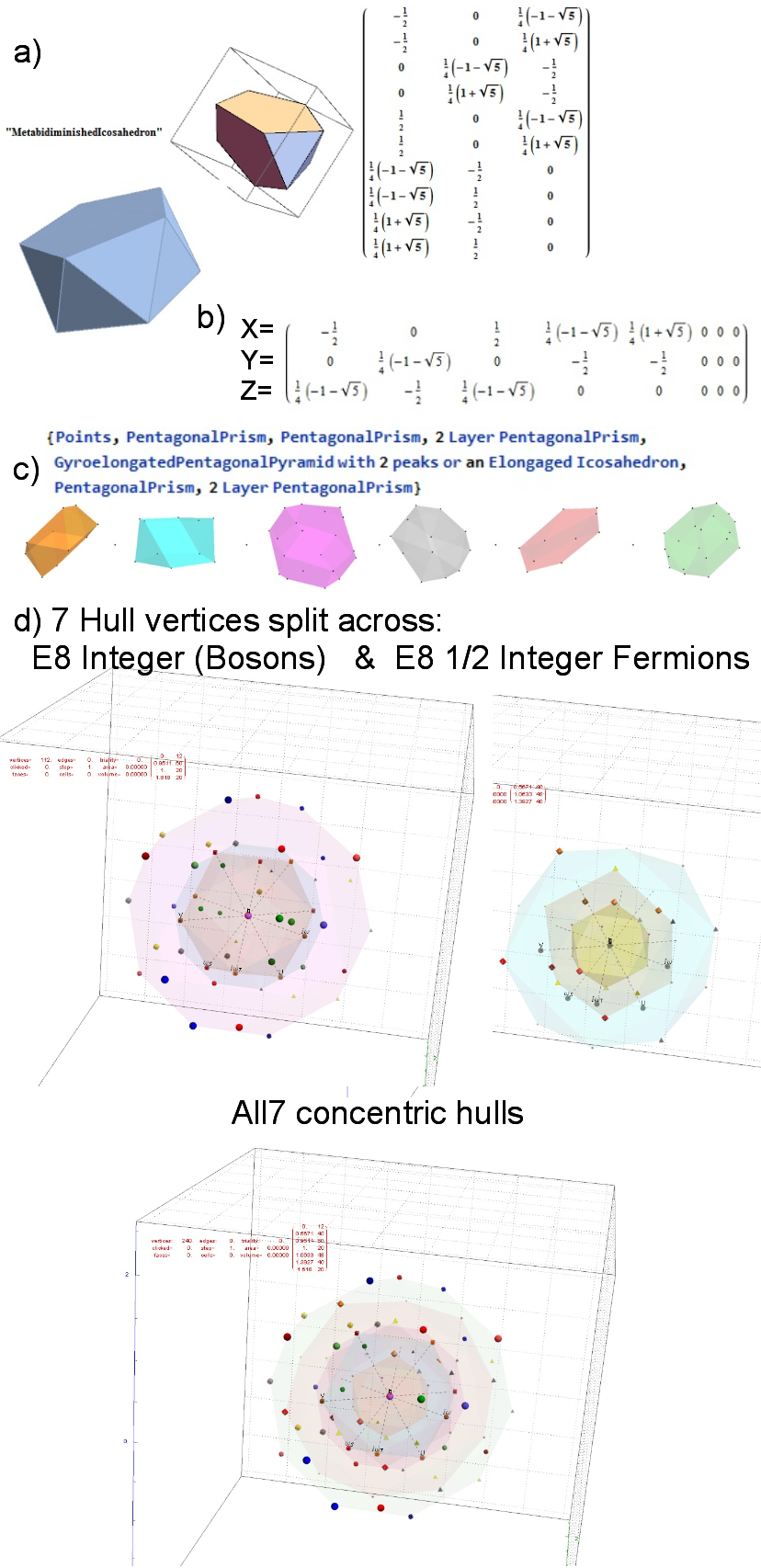FIG. 15: MetabidiminishedIcosahedron (with its negated vertices gives the GyroelongatedPentagonalPyramid) Crystal Projection Prism projecting E8 a) the crystal prism geometry and vertices, b) the selected basis vectors, c) the individual 3D projected concentric hull objects, d) the combined set of concentric objects with progressively increasing transparency and tally of vertex norms.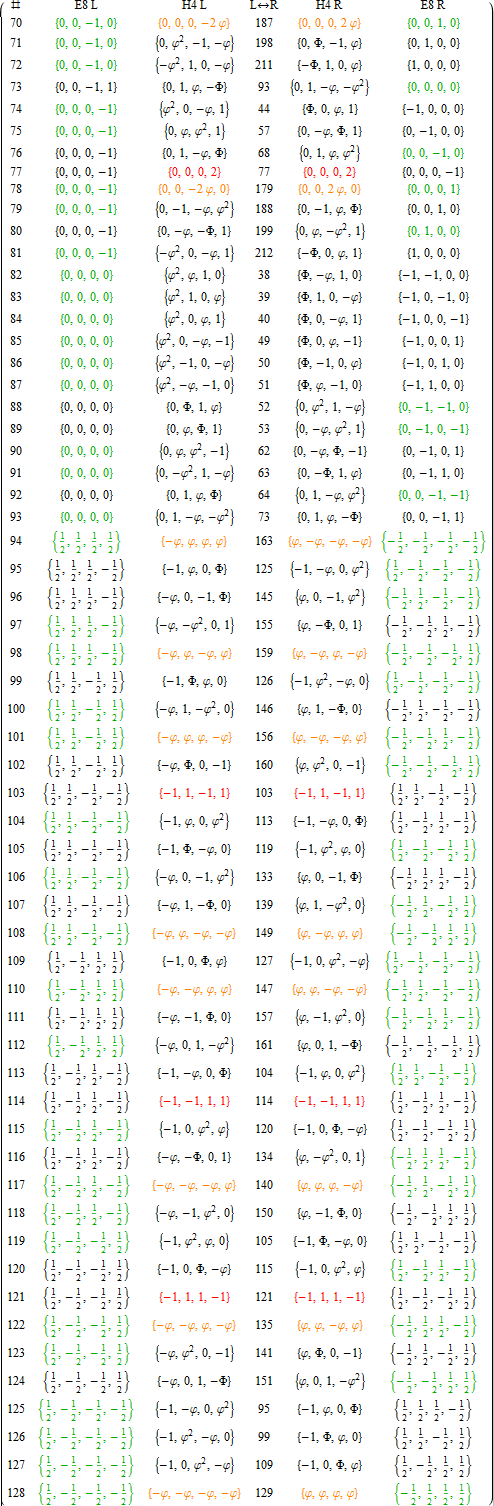APPENDIX A: LIST OF E8 AND ITS ROTATED H4 AND H4Φ VERTICES
This table splits the E8 and its rotated H4 vertices into L/R 4D elements.

1. Table structure
The first column is an SRE E8 vertex index number derived from sorting the E8 vertices by their position based on the 256=2^8 binary pattern from the 9th row of the Pascal triangle (1, 8, 28, 56, 70, 56, 28, 8, 1) and its associated Cl8 Clifford Algebra. This construction is described in more detail in . The odd groups (1,3,5,7,9) with (1,26,70,26,1) elements (respectively) are the 128 1/2 integer vertices. The even groups (2,4,6,8) with (8,56,56,8) elements (respectively) are the 112 integer vertices along with the 16 excluded 8 generator (and 8 anti-generator) vertices (2-9 and 248-255) with permutations of (1; 0; 0; 0; 0; 0; 0; 0) (a.k.a. the 8-Orthoplex), such that they indicate the basis vectors used for projecting the polytope.

Only the first half of the 240 vertices is shown, since the last half is simply the reverse order negation of the vertices
in the first half (e.g. the E8 vertex n=10 has as its’ negation 257-n=247). The middle column labeled L<->R indicates the vertex reference number that contains the same L as the R (and equivalently, the same R as the L, interestingly enough).

2. Table color coding
The E8 L/R columns’ green color-coded elements indicate that the 4D vertex rotates into the smaller H4 600-cell.
Conversely, the E8 L/R columns’ black color-coded elements indicate the 4D vertex rotates into the larger H4Φ
600-cell.

The H4 L/R columns’ red color-coded rows are 24-cell elements that always self-reference and are always members of the scaled up 600-cell H4Φ. The H4 L/R columns’ orange color-coded rows are 24-cell elements that always reference the negated elements in the range of 129-256 and are always members of the smaller 600-cell H4.

# Metatron’s Cube

I was recently asked to create a 3D laser etched crystal of Metatron’s Cube.

The 13 Metatron rings are (or at least can be) taken from the 16 vertices of the tesseract (a 4D geometric figure called an 8-cell) which can be visualized by projecting 4D into 3D as two concentric 3D cubes.

Three of the 16 rings are hidden behind the ring in the front center when projecting the two concentric 3D cubes to 2D (or looking at it from just the correct angle on a corner and ignoring the perspective error introduced by parallax).

There are many more lines in the Metatron cube than from the typical 32 edges of the 8-cell tesseract connecting the vertices. These come from what is called the “complete graph” of 120 edges from the 16 vertices of the 8-cell. The Platonic solids are all contained (or related by duality) within the 3D projection of this complete graph of the 8-cell with large vertex spheres.

Slightly off angle (notice the 3 hidden spheres are now visible):